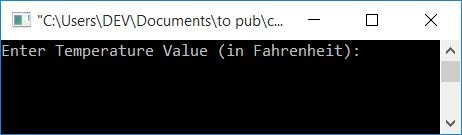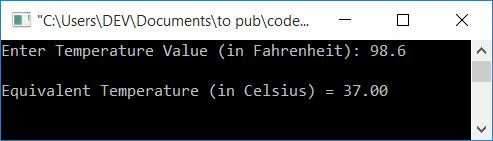# C Program to Convert Fahrenheit to Celsius

In this article, you will learn and get code for the conversion of temperature values from Fahrenheit to Celsius. Before going to the program, let's understand the formula used in its conversion.

### Fahrenheit to Celsius Formula

The Fahrenheit to Celsius formula is:

`celsius = (fahrenheit-32)*(100/180)`

To learn more about this formula, refer to the Celsius to Fahrenheit Formula explanation . There, you will get a complete understanding of how this formula was derived. I know that the title is Celsius to Fahrenheit, but you don't worry about it; you automatically understand the Fahrenheit to Celsius formula after reading that article.

The formula given above can also be written as follows:

`celsius = (fahrenheit-32)/(180/100)`

And as the value of 180/100 is 1.8, the previous formula can be further written as:

`celsius = (fahrenheit-32)/1.8`

Now let's move on to the program written in C for the conversion of Fahrenheit to Celsius.

## Fahrenheit to Celsius in C

The question is: write a program in C that converts temperatures given in degrees Fahrenheit to the equivalent degrees Celsius value. The answer to this question is given below:

```#include<stdio.h>
#include<conio.h>
int main()
{
float fahrenheit, celsius;
printf("Enter Temperature Value (in Fahrenheit): ");
scanf("%f", &fahrenheit);
celsius = (fahrenheit-32)/1.8;
printf("\nEquivalent Temperature (in Celsius) = %0.2f", celsius);
getch();
return 0;
}```

This program is compiled and executed in the Code::Blocks IDE. Let's have a look at the sample run of the above program:Now supply any temperature value (in Fahrenheit), say 98.6, and press the ENTER key to see the following output:Note: The %0.2f format specifier is used to print decimal values up to 2 decimal places.

## Function to convert Fahrenheit to Celsius in C

Let's create another program that does the same job as the previous program. But this time, it is created using a user-defined function. This function takes the value of Fahrenheit as its parameter, converts it into Celsius, and then passes it as its return value.

```#include<stdio.h>
#include<conio.h>
float fahToCelFun(float);
int main()
{
float fahrenheit, celsius;
printf("Enter Temperature Value (in Fahrenheit): ");
scanf("%f", &fahrenheit);
celsius = fahToCelFun(fahrenheit);
printf("\nEquivalent Temperature (in Celsius) = %0.2f", celsius);
getch();
return 0;
}
float fahToCelFun(float fah)
{
return ((fah-32)/1.8);
}```

The output of the above program is identical to the output of the first program given at the beginning of this article.

#### The same program in different languages

C Quiz

« Previous Program Next Program »